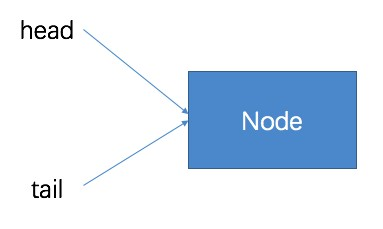jdk1.7.0_79

队列是一种非常常用的数据结构，一进一出，先进先出。

在Java并发包中提供了两种类型的队列，非阻塞队列与阻塞队列，当然它们都是线程安全的，无需担心在多线程并发环境所带来的不可预知的问题。为什么会有非阻塞和阻塞之分呢？这里的非阻塞与阻塞在于有界与否，也就是在初始化时有没有给它一个默认的容量大小，对于阻塞有界队列来讲，如果队列满了的话，则任何线程都会阻塞不能进行入队操作，反之队列为空的话，则任何线程都不能进行出队操作。而对于非阻塞无界队列来讲则不会出现队列满或者队列空的情况。它们俩都保证线程的安全性，即不能有一个以上的线程同时对队列进行入队或者出队操作。

```//ConcurrentLinkedQueue
head = tail = new Node<E>(null);
}```对于入队（插入）操作一共提供了这么2个方法（实际上是一个）：

``` //ConcurrentLinkedQueue#offer
public boolean offer(E e) {
checkNotNull(e);    //入队元素是否为空，不允许Null值入队
final Node<E> newNode = new Node<E>(e);    //将入队元素构造为Node节点
/*tail指向的是队列尾节点，但有时tail.next才是真正指向的尾节点*/
for (Node<E> t = tail, p = t;;) {
Node<E> q = p.next;
if (q == null) {    //此时p指向的就是队列真正的尾节点
if(p.casNext(null, newNode)) {    //cas算法,p.next = newNode
if (p != tail)     //将tail指向队列尾节点
casTail(t, newNode);
return true;
}
}
else if (p == q)
p = (t != (t = tail)) ? t : head;
else
p = (p != t && t != (t = tail)) t : q;
}
}```

offer入队过程如下图所示：
① 队列中没有元素，第一次入队操作：
进入循环体：
t = tail;
p = tail;
q = p.next = null;判断尾节点的引用p是否指向的是尾节点（if(q == null)）->是：
CAS算法将入队节点设置成尾节点的next节点（p.casNext(null, newNode)）
判断tail尾节点指针的引用p是否大于等于1个next节点（if (p != t)）->否
返回true② 队列中有元素，进行入队操作：

1) 第一次循环：
t = tail;
p = tail;
q = p.next = Node1;判断tail尾节点指针的引用p是否指向的是尾节点（if(q == null)）->否
判断tail尾节点指针的引用p是否指向的是尾节点（else if (p == q)）->否
将tail尾节点指针的引用p向后移动（p = (p != t && t != (t = tail)) ? t : q;）->p = Node12) 第二次循环：
t = tail;
p = Node1;
q = p.next = null;判断tail尾节点指针的引用p是否指向真正的尾节点（if(q == null)）->是：
CAS算法将入队节点设置成尾节点的next节点（p.casNext(null, newNode)）
判断tail尾节点指针的引用p是否大于等于1个next节点(if (p != t))->是：
更新tail节点（casTail(t, nextNode)）
返回true对于出队（删除）操作一共提供了这么1个方法：``` //ConcurrentLinkecQueue#poll
public E poll() {
for (;;) {
for (Node<E> h = head, p = h, q;;) {
E item = p.item;
if (item != null && p.casItem(item, null)) {
if (p != h)
updateHead(h, ((q = p.next) != null) ? q : p);
return item;
}
else if ((q = p.next) == null) {
return null;
}
else if (p == q)
else
p = q;
}
}
}```出队（删除）：
1） 第一次循环：
q = null;
item = p.item = null;判断（暂略）
判断（暂略）

2） 第二次循环：
p = q = Node1;
q = Node1;
item = p.item = Node1.item;更新头节点（updateHead(h, ((q = p.next) != null) ? q : p)）
返回item`for (int i = 0, int size = concurrentLinkedQueue.size(); i < size;i++)`